# Designing Flyback Converters Using Peak-Current-Mode Controllers

### 要約

Flyback converter design using MAX17595/MAX17596 is outlined. Design methodology and calculations for components value selection are presented. Continuous conduction mode (CCM) and discontinuous conduction mode (DCM) are treated individually.

### Introduction

This application note describes the methodology of designing flyback converters using the MAX17595/MAX17596 peak-current-mode controllers. Flyback converters may be operated in discontinuous conduction mode (DCM) or continuous conduction mode (CCM). The component choices, stress level in power devices, and controller design vary depending on the operating mode of the converter. Formulas for calculating component values and ratings are also presented.

### DCM Flyback

#### Primary Inductance Selection

In a DCM flyback converter, the energy stored in the primary inductance of the flyback transformer is delivered entirely to the output. The maximum primary-inductance value for which the converter remains in DCM at all operating conditions can be calculated as:Where DMAX is chosen as 0.43 for the MAX17595/MAX17596, VD is the voltage drop of the output rectifier diode on the secondary winding, and FSW is the switching frequency of the power converter. Choose the primary inductance value to be less than LPRIMAX.

#### Duty Cycle Calculation

The accurate value of the duty cycle (DNEW) for the selected primary inductance (LPRI) can be calculated using the following equation: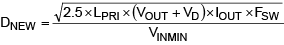#### Turns Ratio Calculation (NS/NP)

Transformer turns ratio (K = NS/NP) can be calculated as:#### Peak/RMS Current Calculation

Primary and secondary RMS currents and primary peak current calculations are needed to design the transformer in switched-mode power supplies. Also, primary peak current is used in setting the current limit. Use the following equations to calculate the primary and secondary peak and RMS currents.

Maximum primary peak current,Maximum primary RMS current,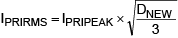Maximum secondary peak current,Maximum secondary RMS current,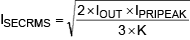For the purpose of current limit setting, the peak current may be calculated as follows:

ILIM = IPRIPEAK × 1.2

#### Primary Snubber Selection

Ideally, the external MOSFET experiences a drain-source voltage stress equal to the sum of the input voltage and reflected voltage across the primary winding during the OFF period of the MOSFET. In practice, parasitic inductors and capacitors in the circuit, such as leakage inductance of the flyback transformer, cause voltage overshoot and ringing in addition to the ideally expected voltage stress. Snubber circuits are used to limit the voltage overshoots to safe levels within the voltage rating of the external MOSFET. The snubber capacitor can be calculated using the following equation:Where LLK is the leakage inductance that can be obtained from the transformer specifications (usually 1% to 2% of the primary inductance).

The power that must be dissipated in the snubber resistor is calculated using the following formula:

PSNUB = 0.833 × LLK × IPRIPEAK² × FSW

The snubber resistor is calculated based on the equation below: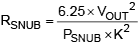The voltage rating of the snubber diode is:

VDSNUB = VINMAX + (2.5 × VOUT/K)

#### Output Capacitor Selection

X7R ceramic output capacitors are preferred in industrial applications due to their stability over temperature. The output capacitor is usually sized to support a step load of 50% of the rated output current in nonisolated applications so that the output voltage deviation is contained to 3% of the rated output voltage. The output capacitance can be calculated as follows:The output capacitor RMS current rating can be calculated as follows: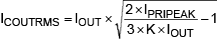Where ISTEP is the load step, TRESPONSE is the response time of the controller, ΔVOUT is the allowable output voltage deviation, and FC is the target closed-loop crossover frequency. FC is chosen to be 1/10 of the switching frequency FSW. For the flyback converter, the output capacitor supplies the load current when the main switch is on, and therefore the output voltage ripple is a function of load current and duty cycle. Use the following equation to calculate the output capacitor ripple:Where IOUT is load current and DNEW is the duty cycle at minimum input voltage.

#### Input Capacitor Selection

The MAX17595 is optimized to implement offline AC-DC converters. In such applications, the input capacitor must be selected based on either the ripple due to the rectified line voltage, or based on holdup-time requirements. Holdup time can be defined as the time period over which the power supply should regulate its output voltage from the instant the AC power fails. The MAX17596 is useful in implementing low-voltage DC-DC applications where the switching-frequency ripple must be used to calculate the input capacitor. In both cases, the capacitor must be sized to meet RMS current requirements for reliable operation.

#### Capacitor Selection Based on Switching Ripple (MAX17596)

For DC-DC applications, X7R ceramic capacitors are recommended due to their stability over the operating temperature range. The effective series resistance (ESR) and effective series inductance (ESL) of a ceramic capacitor are relatively low, so the ripple voltage is dominated by the capacitive component. For the flyback converter, the input capacitor supplies the current when the main switch is on. Use the following equation to calculate the input capacitor for a specified peak-to-peak input switching ripple (VIN_RIP):The input capacitor RMS current in low-voltage DC-DC applications can be calculated as follows:#### Capacitor Selection Based on Rectified Line Voltage Ripple (MAX17595)

For the flyback converter, the input capacitor supplies the input current when the diode rectifier is off. The voltage discharge on the input capacitor, due to the input average current, should be within the limits specified.

Assuming 25% ripple present on input DC capacitor, the input capacitor can be calculated as follows:Where

η = typical efficiency at VAC,MIN and ILOAD

VIN,PK = √2 × VAC,MIN = peak voltage at minimum input AC voltage.

#### Capacitor Selection Based on Holdup Time Requirements (MAX17595)

For a given output power (PHOLDUP) that needs to be delivered during holdup time (THOLDUP), the DC bus voltage at which the AC supply fails (VINFAIL), and the minimum DC bus voltage at which the converter can regulate the output voltages (VINMIN), the input capacitor (CIN) is estimated as:The input capacitor RMS current for AC-DC applications can be calculated as: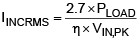#### External MOSFET Selection

MOSFET selection criteria include maximum drain voltage, peak/RMS current in the primary, and the maximum allowable power dissipation of the package without exceeding the junction temperature limits. The voltage seen by the MOSFET drain is the sum of the input voltage, the reflected secondary voltage on the transformer primary, and the leakage inductance spike. The MOSFET’s absolute maximum VDS rating must be higher than the worst-case drain voltage:The drain current rating of the external MOSFET is selected to be greater than the worst-case peak current limit setting.

#### Secondary Diode Selection

Secondary-diode-selection criteria includes the maximum reverse voltage, average current in the secondary, reverse recovery time, junction capacitance, and the maximum allowable power dissipation of the package. The voltage stress on the diode is the sum of the output voltage and the reflected primary voltage. The maximum operating reverse-voltage rating must be higher than the worst-case reverse voltage:

VSECDIODE = 1.25 × (K × VINMAX + VOUT)

The current rating of the secondary diode should be selected so that the power loss in the diode (given as the product of forward-voltage drop and the average diode current) should be low enough to ensure that the junction temperature is within limits. Select fast-recovery diodes with a recovery time less than 50ns, or Schottky diodes with low junction capacitance.

#### Error Amplifier Compensation Design

For nonisolated designs, output voltage feedback and the loop compensation network are connected as shown in Figure 1.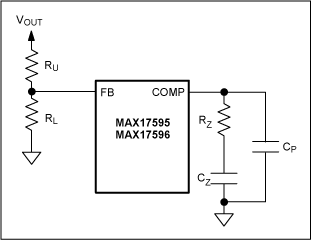The loop compensation values are calculated as:Where: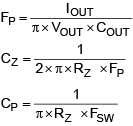FSW is the switching frequency.

### CCM Flyback

#### Transformer Turns Ratio Calculation (K = NS/NP)

The transformer turns ratio can be calculated using the following formula:Where DMAX is the duty cycle assumed at minimum input (0.43 for the MAX17595/MAX17596).

#### Primary Inductance Calculation

Calculate the primary inductance based on the ripple:Where DNOM, the nominal duty cycle at nominal operating DC input voltage VINNOM, is given as:The output current, down to which the flyback converter should operate in CCM, is determined by selection of β in Equation 27. For example, β should be selected as 0.15 so that the converter operates in CCM down to 15% of the maxim output load current. The ripple in the primary current waveform is a function of the duty cycle; maximum ripple occurs at the maximum DC input voltage. Therefore, the maximum (worst-case) load current down to which the converter operates in CCM occurs at the maximum operating DC input voltage. VD is the forward drop of the selected output diode at maximum output current.

#### Peak and RMS Current Calculation

Primary and secondary RMS currents and primary peak current calculations are needed to design the transformer in switched-mode power supplies. Also, primary peak current is used in setting the current limit. Use the following equations to calculate the primary and secondary peak and RMS currents.

Maximum primary peak current: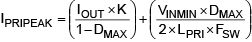Maximum primary RMS current: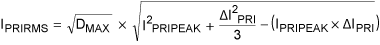Where ΔIPRI is the ripple current in the primary current waveform, and is given by:Maximum secondary peak current:

ISECPEAK = IPRIPEAK/K

Maximum secondary RMS current:Where ΔISEC is the ripple current in the secondary current waveform, and is given by: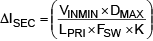For the purpose of current-limit setting, the peak current can be calculated as follows:

ILIM = IPRIPEAK × 1.2

#### Primary RCD Snubber Selection

The design procedure for primary RCD snubber selection is identical to that outlined in the DCM Flyback section.

#### Output Capacitor Selection

X7R ceramic output capacitors are preferred in industrial applications due to their stability over temperature. The output capacitor is usually sized to support a step load of 50% of the rated output current in nonisolated applications so that the output-voltage deviation is contained to 3% of the rated output voltage. The output capacitance can be calculated as:The output capacitor RMS current rating can be calculated as follows:Where ISTEP is the load step, TRESPONSE is the response time of the controller, ΔVOUT is the allowable output voltage deviation, and FC is the target closed-loop crossover frequency. FC is chosen to be less than 1/5 of the worst-case (lowest) RHP zero frequency FRHP. The right half-plane zero frequency is calculated as follows:For the CCM flyback converter, the output capacitor supplies the load current when the main switch is on, and therefore, the output-voltage ripple is a function of load current and duty cycle. Use Equation 40 to estimate the output-voltage ripple: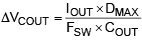#### Input Capacitor Selection (MAX17596)

Use Equation 41 to calculate the input capacitor for a specified peak-to-peak input switching ripple (VIN_RIP) in low-voltage DC-DC converters operating in CCM mode.#### Error Amplifier Compensation Design

For nonisolated designs, the output voltage feedback and loop compensation network are connected as shown in Figure 1.

In the CCM flyback converter, the primary inductance and the equivalent load resistance introduces a right half-plane zero at the following frequency:The loop compensation values are calculated as:Where FP, the pole due to output capacitor and load, is given by:The above selection of RZ sets the loop-gain crossover frequency (FC, where the loop gain equals 1) equal to 1/5 the right-half plane zero frequency.

FC ≤ FZRHP/5

With the control loop zero placed at the load pole frequency:With the high-frequency pole placed at half the switching frequency:### Isolated Flyback with Optocoupler Feedback

Optocoupler feedback is used in isolated flyback converter designs for precise control of isolated output voltage. This section describes the different configurations of a controller and outlines a general procedure to calculate compensating network component values. Flyback converter designs, operating in both DCM and CCM, are covered.

The overall scheme of optocoupler feedback is shown in Figure 2.Use RFB = 470Ω (typical), for an optocoupler transistor current of 1mA. Select R1 = 49.9kΩ and R2 = 22kΩ (typical values), to use the full range of available COMP voltage. U3 is a low-voltage adjustable shunt regulator with a 1.24V reference voltage. Calculate RLED using Equation 48, based on output voltage VOUT.

RLED = 400 × CTR × (VOUT - 2.7)Ω

The bandwidth of typical optocouplers limits the achievable closed loop bandwidth of opto-isolated converters. And in CCM flyback designs, the presence of right-half-plane (RHP) zero limits the practical bandwidth of the closed-loop system. Considering these limitations, the closed-loop crossover frequency may be chosen, at the nominal input voltage as follows:

ƒC = 5kHz, for DCM designs

Or ƒC = ƒZRHP/10, for CCM designs, limited to ƒC = 5kHz

Closed-loop compensation values are designed based on the open-loop gain at the desired crossover frequency, ƒC. The open-loop gains in DCM and CCM, at ƒC, are calculated using the following expressions.

Or ƒC = ƒZRHP/10, for CCM designs, limited to ƒC = 5kHz, for DCM designs

And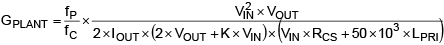, for CCM designs

Where

K = NS/NP is the transformer turns ratio

ƒP = IOUT/(π × VOUT × COUT), for DCM designs

And, for CCM designs

Three controller configurations are suggested, based on open-loop gain and the value of RLED. For typical designs, the current transfer ratio (CTR) of the optocoupler designs can be assumed to be unity. It is known that the comparator and gate driver delays associated with the input voltage variations affects the optocoupler CTR. Depending on the optocopler selected, variations in CTR causes wide variations in bandwidth of the closed-loop system across the input-voltage operating range. It is recommended to select an optocoupler with less CTR variations across the operating range.

Configuration 1:The schematic for this controller configuration is depicted in Figure 3.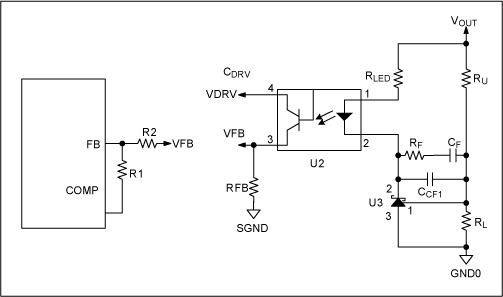Configuration 2:The schematic for this controller configuration is depicted in Figure 4.Configuration 3: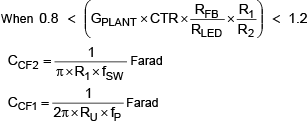The schematic for this controller configuration is depicted in Figure 5.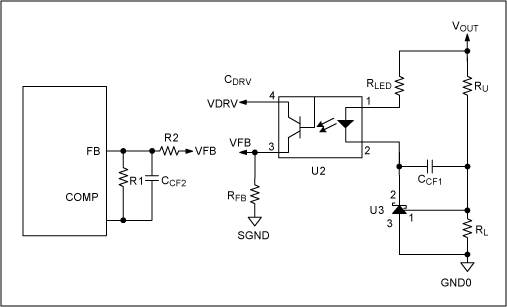### Bias Winding Supply Configuration

The MAX17595 is implemented with a 20V VIN UVLO wake-up level with 13V hysteresis to optimize the size of bias capacitor. A simple RC circuit is used to start up the MAX17595. To sustain the operation of the circuit, the input supply to the IC is bootstrapped through diode D2 as shown in Figure 6 (refer to the MAX17595–7 data sheet to design the startup network).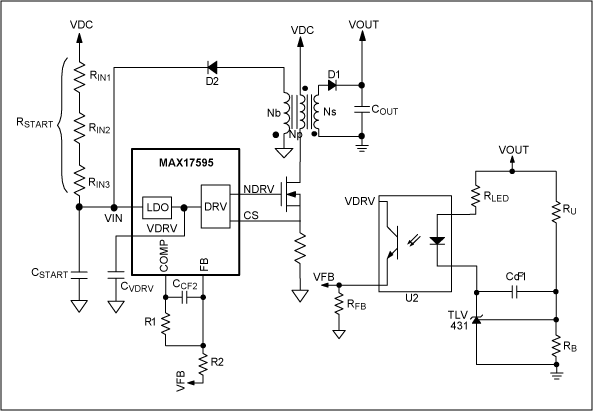#### Turns Ratio Calculation (NB/NP)

The transformer turns ratio (KB = NB/NP) can be calculated as follows:#### Bias Capacitor (CSTART) Calculation

In isolated applications where a bias winding configuration is used to power up MAX17595/MAX17596, CSTART can be calculated as follows:

CSTART = 0.75 × (CDRV + 0.1 × IIN × TSS + 0.04 × TSS × QG × FSW)

#### Feedback Potential Divider Selection (RU, RB)

For all the applications that use a startup network to bias IN pin during the power-up sequence, calculate the feedback potential divider using the following formulas.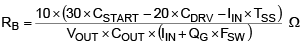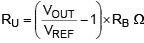Where VREF is the reference set by the secondary side controller (e.g., VREF = 1.24V for TLV431)

CSTART is the startup capacitor, CDRV is the cumulative capacitor used at the DRV pin, IIN is the MAX17595 quiescent current, TSS is the soft-start time, VOUT is the output voltage, COUT is the output capacitor used, and QG is the gate charge of the primary n-channel MOSFET.

The bias winding configuration is not needed in low-voltage DC-DC applications where the input voltage can be directly used for IC supply, as shown in Figure 7. Using the input supply directly for the IC eliminates the external RC startup network and bias winding circuit. The MAX17596 is optimized for such low-voltage DC-DC applications with UVLO VIN wake-up level of 4.1V (typ) with 200mV hysteresis. In such applications where bias winding is not used, the feedback potential divider may be chosen as follows:

Choose RB = 10kΩ (typ)### Typical Operating Circuit### Design Calculations for the MAX17595-Based Isolated DCM Flyback Converter

#### Technical Specifications

Input voltage range: 85VAC to 265VAC
Output voltage: 15V
Rated output current: 1.5A
Switching frequency: 120kHz
Operating mode: Discontinuous Conduction Mode (DCM)

1. Primary inductance selection
In offline applications, the DC bus voltage varies from 120VDC to 375VDC. But the actual minimum input operating voltage depends on the 100Hz ripple present on the DC bus capacitor. In this application, the ripple is assumed to be 30V and hence the minimum DC input to the converter is 90V.
2.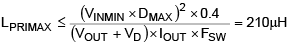Where VINMIN = 90V, DMAX = 0.43, VD = 0.8V

Select primary inductance LPRI = 190µH to account for 10% tolerance on primary inductance.

3. Maximum duty cycle calculation with selected LPRI
4.5. Turns ratio calculation (K = NS/NP)
6.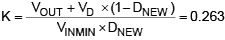7. Peak/RMS current calculation
8.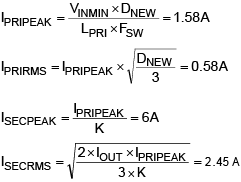9. Primary snubber selection
10.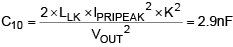Where leakage inductance LLK = 1.9µH (1% of LPRI)

Considering the derating of the snubber capacitor, select C10 = 3.3nF.

PR18 = 0.833 × LLK × IPRIPEAK² × FSW = 0.47W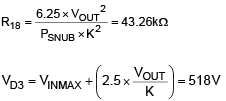11. Output capacitor selection
12. FC = 5kHz, typical bandwidth at nominal voltage for isolated applications

ISTEP = 0.25 × IOUT = 0.375A (25% of ILOAD, typical for isolated applications)

ΔVOUT = 450mV (3% of VOUT, typical)Actual output capacitance used in the application is:

C13,14,15,16 = 30µF (with 22µF/25V × 4 capacitors after derating)

Note: Capacitor values change with temperature and applied voltage. Refer to capacitor data sheets to select capacitors that guarantee the required output capacitance across the operating range. For design calculations, use the worst-case derated value of capacitance, based on temperature range and applied voltage.13. Input capacitor selection based on 100Hz ripple on DC bus voltage
14. η = 0.85 (typical efficiency)

PLOAD = 15 × 1.5 = 22.5W

VIN,PK = √2 × 85 = 120V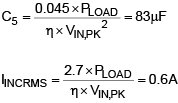15. External MOSFET selection
16.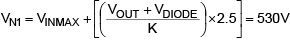17. Secondary diode selection
18. VD4 = 1.25 × (K × VINMAX + VOUT) = 140V

19. Bias winding supply configuration
20. For offline applications, bias supply configuration as shown in Figure 6 is used to power up the MAX17595.

Use VBIAS = 12V, VD2 = 0.8V, VD1 = 0.8V, K = 0.24, CDRV = 1µF, IIN = 2mA, QG = 35nC (STB11NM80), R1 = 49.9kΩ, R2 = 22kΩ for the following calculations.

Select soft-start time TSS = 12ms (refer to the MAX17595/MAX17596/MAX17597 data sheet for programming soft-start time).

21. Output-voltage soft-start time calculation
22. In isolated designs, the output-voltage soft-start time depends on the values of TSS, R1, and R2 and can be calculated as follows:Note: The above equation provides an approximate output-voltage soft-start time. Due to the presence of the optocircuit, the actual soft-start may be different from the programmed soft-start time. It is recommended to adjust the soft-start capacitor to get the required soft-start time.

23. Bias turns ratio selection (KB = NB/NP)
24.25. Startup capacitor selection
26. C9 = 0.75 × (CDVR + 0.1 × IIN × TSS + 0.04 × TSS × QG × FSW) = 4µF

Note: Usually the bias voltage is in the range of 12V to 20V, so it is suggested to consider the derating of the startup capacitor. Improper selection of the CSTART, RL, and RU may result in an unnecessary power up sequence if IN supply falls below the UVLO lower threshold during circuit operation.

27. Feedback potential divider selection
28.Select R29 = 221Ω29. Isolated flyback with opto-isolated feedback compensation design
30. Choose VIN = 325VDC, R1 = 49.9kΩ, R2 = 22kΩ, RFB = 470Ω, CTR = 1 (all typical values)

RCS = 0.2Ω

R26 = 400 × CTR × (VOUT - 2.7)Ω = 4.9kΩ

Choose fC = 5kHz (typical, at nominal input voltage of 325VDC)Since the loop gain is less than 1.2, the third configuration shown in the isolated compensation design should be used for this application.Select C17 = 68nFSelect C4 = 56pF

 Designation Qty Description C1 1 0.1µF 20% 275VAC X2 plastic film capacitor (17mm x 5mm) Panasonic ECQ-U2A104ML C4 1 56pF 5% 50V C0G ceramic capacitor (0603) Murata GRM1885C1H560J C5 1 100µF 20% 450V aluminium electrolytic capacitor (25mm diameter) Panasonic ECO-S2GP101CA C6, C21 2 0.47µF 10% 25V X7R ceramic capacitors (0603) Murata GRM188R71E474K C7 1 0.1µF 10% 16V X7R ceramic capacitor (0603) Murata GRM188R71C104K C8 1 1µF 10% 25V X7R ceramic capacitor (0603) Murata GRM188R71E105K C9 1 4.7µF 10% 50V X7R ceramic capacitor (1206) Murata GRM31CR71H475K C10 1 3300pF 10% 250V X7R ceramic capacitor (0805) Murata GRM21AR72E332K C11 1 1000pF 10% 50V X7R ceramic capacitor (0603) Murata GRM188R71H102K C13, C14, C15, C16 4 22µF 10% 25V X7R ceramic capacitors (1210) Murata GRM32ER71E226K C17 1 68nF 10% 50V X7R ceramic capacitor (0603) TDK C1608X7R1H683K D1 1 600V 1.5A bridge rectifier (DF-S) Diodes Inc. DF1506S D2 1 100V 300mA fast switching diode (SOD-123) Diodes Inc. 1N4148W-7-F D3 1 800V 1A ultra-fast rectifier (SMA) Diodes Inc. US1K-TP D4 1 200V 6A ultra-fast recovery rectifier (PowerDI 5) Diodes Inc. PDU620-13 L1 1 6.8mH 0.8A line filter (13mm x 10mm) Panasonic® ELF15N008A N1 1 800V 11A N-channel MOSFET (D2PAK) ST Micro STB11NM80T4 R1 1 10Ω 2A NTC thermistor (5mm) EPCOS B57153S0100M000 R2, R3, R4 3 549kΩ 1% resistors (1206) R5 1 19.8kΩ 1% resistor (0603) R6 1 4.99kΩ 1% resistor (0603) R9 1 82.5kΩ 1% resistor (0603) R12 1 49.9kΩ 1% resistor (0603) R13 1 22kΩ 1% resistor (0603) R14, R15, R16 3 402kΩ 1% resistors (1206) R18 2 100kΩ 5% resistors (1206) Panasonic ERJ-P08J104V R19 1 10Ω 1% resistor (0603) R20 1 100Ω 1% resistor (0603) R21 1 0.2Ω 1% resistor (1206) Panasonic ERJ-8BSFR20V R22 1 470Ω 1% resistor (0603) R26 1 4.99kΩ 1% resistor (0603) R28 1 2.49kΩ 1% resistor (0603) R29 1 221Ω 1% resistor (0603) T1 1 180µH, 0.8A, 1:0.24:0.2 transformer (EFD25) Coilcraft® MA5475-AL U1 1 Peak-current-mode controller for flyback regulator (16-TQFN 3mm x 3mm x 0.8mm) Maxim MAX17595ATE+ U2 1 Phototransistor (6-DIP) Avago™ 4N35-300E U3 1 Shunt regulator 1.24V 0.5% (SOT-23-3) Diodes Inc. TLV431BFTA

NOTE: The design methodology for isolated fly back converter using MAX17596 is same as the MAX17595.